### Biconnected Components and Articulation Points

A biconnected component of a graph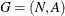is a connected subgraph that cannot be broken into disconnected pieces by deleting any single node (and its incident links). An articulation point is a node of a graph whose removal would cause an increase in the number of connected components. Articulation points can be important when you analyze any graph that represents a communications network. Consider an articulation point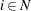which, if removed, disconnects the graph into two components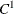and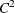. All paths in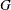between some nodes inand some nodes inmust pass through node. In this sense, articulation points are critical to communication. Examples of where articulation points are important are airline hubs, electric circuits, network wires, protein bonds, traffic routers, and numerous other industrial applications.

In PROC OPTNET, you can find biconnected components and articulation points of an input graph by invoking the BICONCOMP statement. This algorithm works only with undirected graphs.

The results for the biconnected components algorithm are written to the output links data set that is specified in the OUT_LINKS= option in the PROC OPTNET statement. For each link in the links data set, the variable biconcomp identifies its component. The component identifiers are numbered sequentially starting from 1. The results for the articulation points are written to the output nodes data set that is specified in the OUT_NODES= option in the PROC OPTNET statement. For each node in the nodes data set, the variable artpoint is either 1 (if the node is an articulation point) or 0 (otherwise).

The algorithm used by PROC OPTNET to compute biconnected components is a variant of depth-first search (Tarjan 1972). This algorithm runs in time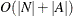and therefore should scale to very large graphs.

#### Biconnected Components of a Simple Undirected Graph

This section illustrates the use of the biconnected components algorithm on the simple undirected graphshown in Figure 2.10.

Figure 2.10: A Simple Undirected Graph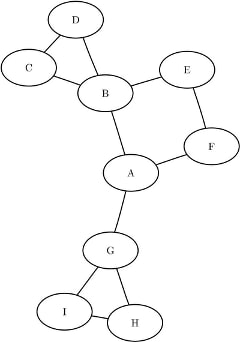The undirected graphcan be represented by the links data set LinkSetInBiCC as follows:

data LinkSetInBiCC;
input from $to$ @@;
datalines;
A B  A F  A G  B C  B D
B E  C D  E F  G I  G H
H I
;


The following statements calculate the biconnected components and articulation points and output the results in the data sets LinkSetOut and NodeSetOut:

proc optnet
out_nodes  = NodeSetOut;
biconcomp;
run;


The data set LinkSetOut now contains the biconnected components of the input graph, as shown in Figure 2.11.

Figure 2.11: Biconnected Components of a Simple Undirected Graph

from to biconcomp
A B 2
A F 2
A G 4
B C 1
B D 1
B E 2
C D 1
E F 2
G I 3
G H 3
H I 3

In addition, the data set NodeSetOut contains the articulation points of the input graph, as shown in Figure 2.12.

Figure 2.12: Articulation Points of a Simple Undirected Graph

node artpoint
A 1
B 1
F 0
G 1
C 0
D 0
E 0
I 0
H 0

The biconnected components are shown graphically in Figure 2.13 and Figure 2.14.

Figure 2.13: Biconnected Componentsand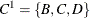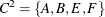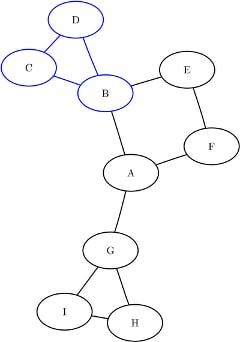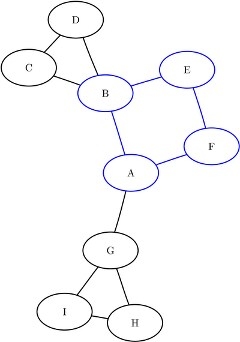Figure 2.14: Biconnected Components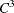and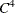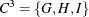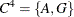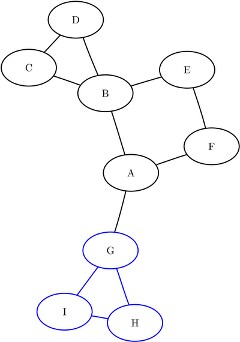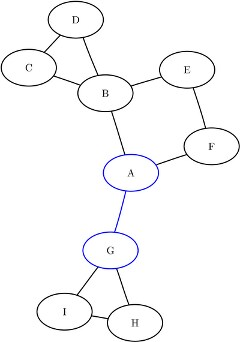For a more detailed example, see Articulation Points in a Terrorist Network.